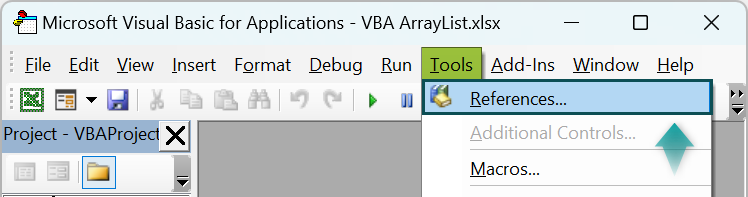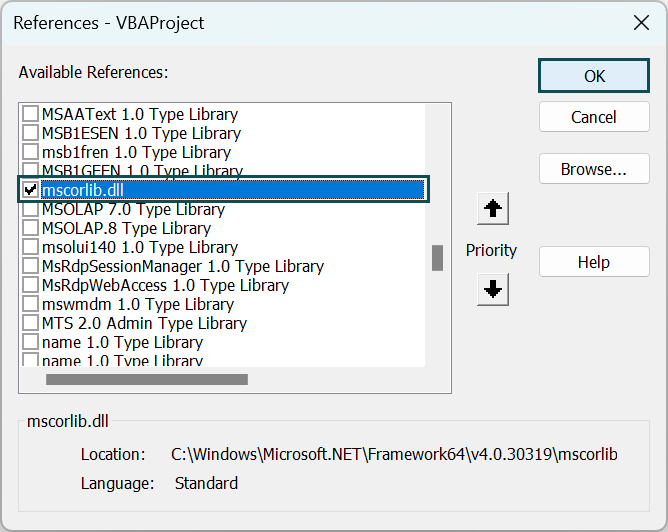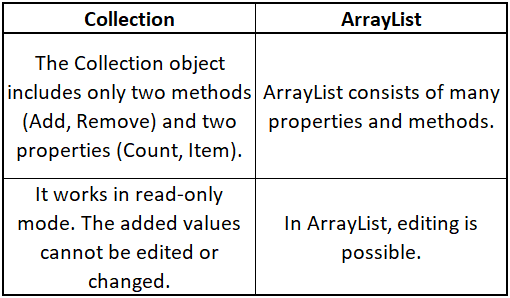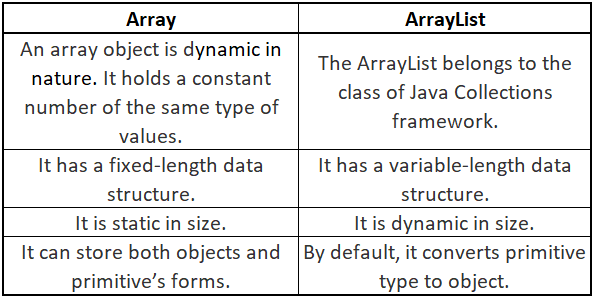# VBA ArrayListArticle byExcelMojo Team## What is Excel VBA ArrayList?

An ArrayList is a VBA object that stores various values and information such as numbers, strings, dates, arrays, ranges, variables, and objects. To use ArrayList in VBA, we must add it in a library “mscorlib.dll.”

Let us see a simple example of a VBA Arraylist to add values. Insert a new module and write the below code:

Run the code using the Run option in the VB ribbon or press F5 and see the added values in the message box as shown below. Press “OK” after every result to see another value.

###### Key Takeaways
• VBA ArrayList is an object used to store a heterogenous collection of data.
• It is pretty similar to the collection object in Excel. However, collection object has many limitations.
• We need to add the ‘mscorlib.dll’ library to work with ArrayList in VBA. For this, click on Tools in the VB ribbon and select References. In the different “Reference” library options, choose ‘mscorlib.dll’ and press Ok.
• It has an in-built sorting function, making them preferable to other storing objects like Collection, Arrays, Dictionary, etc.
• VBA ArrayList alternative is the List(of an object) function in VBA.
• Index values are used for the identification of list values.

### More on Array List

Compared to normal arrays, ArrayList is dynamic. Moreover, VBA ArrayList length is variable compared to the fixed-length data structure in Excel arrays.

To add the library “mscorlib.dll” for working with ArrayList in VBA:

1. Open a VB Editor window, click “Tools” in the VB ribbon, and select “References” to open different libraries list supporting VBA.2. Scroll down and select “mscorlib.dll” and press OK.Once this library is included, we can use ArrayList in our code.

### Examples

We can learn how to store values by adding or removing values in VBA using ArrayList.

#### Example #1

To add text values using ArrayList in VBA. Below are the steps for the same.

• Step 1: Start the code with a sub-procedure. To include an ArrayList into the VBA function as an object, declare a variable called “Values” as an “ArrayList.”
• Step 2: Now, set a new instance for the ArrayList. For this, we use the SET statement and “New” to create an instance of the ArrayList object. The below statement does that:

Set Values = New ArrayList

• Step 3: To add values to the ArrayList, use the “Add” property in which the values are added. These values are internally stored for the index  0,1,2,3 etc., as done for a traditional array.

• Step 4: To see the stored values, use MsgBox to print them into it. Here, like in traditional arrays, we refer to the array values as ArrayName(0), Arrayname(1), etc. You can see the entire code below.

vbNewLine prints the values in a new line.

Sub VBAArrayList_Ex1()
Dim Values As ArrayList
Set Values = New ArrayList
MsgBox Values(0) & vbNewLine & Values(1) & vbNewLine & Values(2) & vbNewLine & Values(3)
End Sub

• Step 5: Run the code using the F5 key and see the stored values partitioned in the message box as shown below:

#### Example #2

In this example, we will see the use of the VBA ArrayList to remove the values from the list of values. Below are the steps to understand how it is done:

• Step 1: Write the sub-procedure in the VB editor and define the ArrayList object.
• Step 2: Now, add values to the array list using the .Add method as shown below:
• Step 3: Write a command to insert ‘Value6’ at the index position 2 and remove the ”Value2.“ For this, we add the following statements to the code.

MyList.Insert 2, “Value6”
MyList.Remove “Value2”

• Step 4: After this, remove the value at the index position 2 and two consecutive values starting from there, i.e., for index positions 2 and 3.

Here, the RemoveAt method removes an element at a specific index.

The RemoveRange removes multiple elements without using a loop. You must specify the starting position and the number of items to remove—for instance, arraylistname.RemoveRange 1,2 removes two elements starting from index 1.

Sub RemoveExample()
Dim MyList As New ArrayList
MyList.Insert 2, “Value6”
MyList.Remove “Value2”
MyList.Remove “Value”
MyList.RemoveAt 2
MyList.RemoveRange 3, 2
End Sub

• Step 5: Repeat the array list to show what is left of the array and the current index position using the For loop in VBA. Here, the message box “MsgBox MyList(N) & ” Index ” & N” shows the Nth value and its index value. The final code is as below:

Code:

Sub RemoveExample()
Dim MyList As New ArrayList
MyList.Insert 2, “Value6”
MyList.Remove “Value2”
MyList.Remove “Value”
MyList.RemoveAt 2
MyList.RemoveRange 3, 2
For N = 0 To MyList.Count – 1
MsgBox MyList(N) & ” Index ” & N
Next N
End Sub

• Step.6: Run the code and see the result below. Press Ok after every value to see the next value.

### Important Things to Note

• An ArrayList is one of the data structures in Excel and is dynamic compared to normal Arrays in VBA (Static).
• Arraylist is not a part of VBA; it is added as a library for use in VBA.
• VBA ArrayList library ‘mscorlib.dll’ is added with the .NET framework.
• To work with an ArrayList in VBA, one should have a minimum of .NET 3.5 or higher versions of the .NET framework.
• VBA ArrayList Sort function sorts the inserted values in ascending or descending orders.
• To Fix Compile Error: “User-defined Type Not Defined”, check for the .NET framework version in your windows, and if not available, add the desired .NET framework by using the following steps:
• Go to Tools →Reference.
• Select the library “Microsoft Scripting Runtime.”

1. What is the difference between ArrayList and collection in excel VBA?

Below are the differences between them.2. What is the maximum size of ArrayList in VBA?

VBA ArrayList has sufficient memory to store many elements. The maximum size is 2,147,483,647.

3. What is the equivalent of ArrayList in VBA?

The equivalent of ArrayList in VBA is the in-built Collection object. The Collection object is very similar to the ArrayList object. However, it possesses certain limitations. We can edit the value in Arraylist compared to the Collection object.

4. What is the difference between ArrayList and array in Visual Basic?This has been a guide to VBA ArrayList. Here we learn how to enable & use ArrayList in Excel VBA, difference from array, along with step-by-step examples. You can learn more from the following articles –